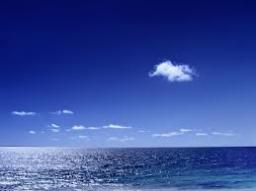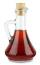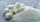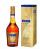# Sea water

Mixing 62 kg of sea water with 84 kg rainwater is created water containing 3.1% salt. How many percent sea water contains salt?

Correct result:

x =  7.3 %

#### Solution:We would be pleased if you find an error in the word problem, spelling mistakes, or inaccuracies and send it to us. Thank you!Tips to related online calculators
Do you have a linear equation or system of equations and looking for its solution? Or do you have quadratic equation?
Tip: Our volume units converter will help you with the conversion of volume units.
Tip: Our Density units converter will help you with the conversion of density units.

## Next similar math problems:

• VinegarWhat percentage vinegar we get if we mix 1 if dm³ eight percent vinegar with 1.5 dm³ six percent vinegar?
• Two workersTwo workers should fulfill certain task together for 5 days. If the first worker increased their performance twice and second twice fell, it took them just four days. For how many days would handle the entire task first worker himself?
• PoolIf water flows into the pool by two inlets, fill the whole for 19 hours. The first inlet filled pool 5 hour longer than the second. How long pool take to fill with two inlets separately?
• SaltSea water containing 5% salt. How many kg of fresh water necessary to pour into 40 kg of sea water so that the salinity fell to 2%?
• Fruit juiceFruit juice contains 37.5% sugar. How many percents of sugar will be in the flavored mineral that we prepare from 100 grams of fruit juice and 1.4 liters of mineral? (1 liter = 1 kg)
• BoatsThree-quarters of boats are white, 1/7 are blue and 9 are red. How many boats do we have?
• Lookout towerHow high is the lookout tower? If each step was 3 cm lower, there would be 60 more of them on the lookout tower. If it was 3 cm higher again, it would be 40 less than it is now.
• Apples 2Dried apples contain 15% water. Fresh apples contain 80% water. How many kg of apples we need buy in order to get 3 kg of dried apples?
• RingThe ring made from gold and copper alloy has a weight of 14.5 g and a volume of 1.03 cm3. How much gold and how much copper it contains? The metal densities are Au 19.3 g/cm³ and Cu 8.94 kg·dm-3
• AlcoholHow many 55% alcohol we need to pour into 14 liters 75% alcohol to get p3% of the alcohol? How many 65% alcohol we get?
• AlcoholHow many cubic centimeters of 96% alcohol is needed to obtain 0.75 liter of 40% alcohol?
• Digit sumThe digit sum of the two-digit number is nine. When we turn figures and multiply by the original two-digit number, we get the number 2430. What is the original two-digit number?
• Conical bottleWhen a conical bottle rests on its flat base, the water in the bottle is 8 cm from it vertex. When the same conical bottle is turned upside down, the water level is 2 cm from its base. What is the height of the bottle?
• PlotThe length of the rectangle is 8 smaller than three times the width. If we increase the width by 5% of the length and the length is reduced by 14% of the width, the circumference of rectangle will be increased by 30 m. What are the dimensions of the recta
• Cuboid wallsCalculate the volume of the cuboid if its different walls have area of 195cm², 135cm² and 117cm².
• Triangular prismThe base of the perpendicular triangular prism is a rectangular triangle with a hypotenuse of 10 cm and one leg of 8 cm. The prism height is 75% of the perimeter of the base. Calculate the volume and surface of the prism.
• VintnerHow high can vintner fill keg with crushed red grapes if these grapes occupy a volume of 20 percent? Keg is cylindrical with a diameter of the base 1 m and a volume 9.42 hl. Start from the premise that says that fermentation will fill the keg (the number